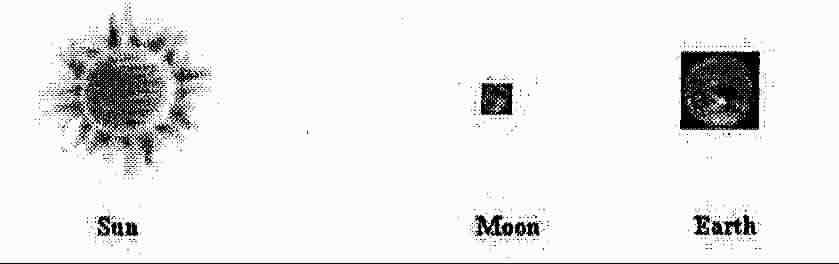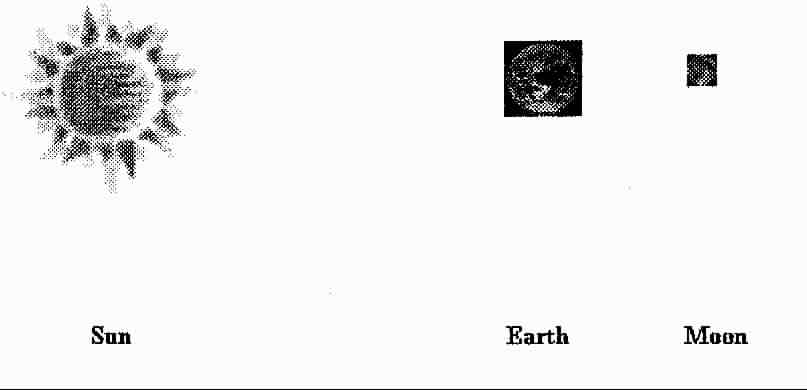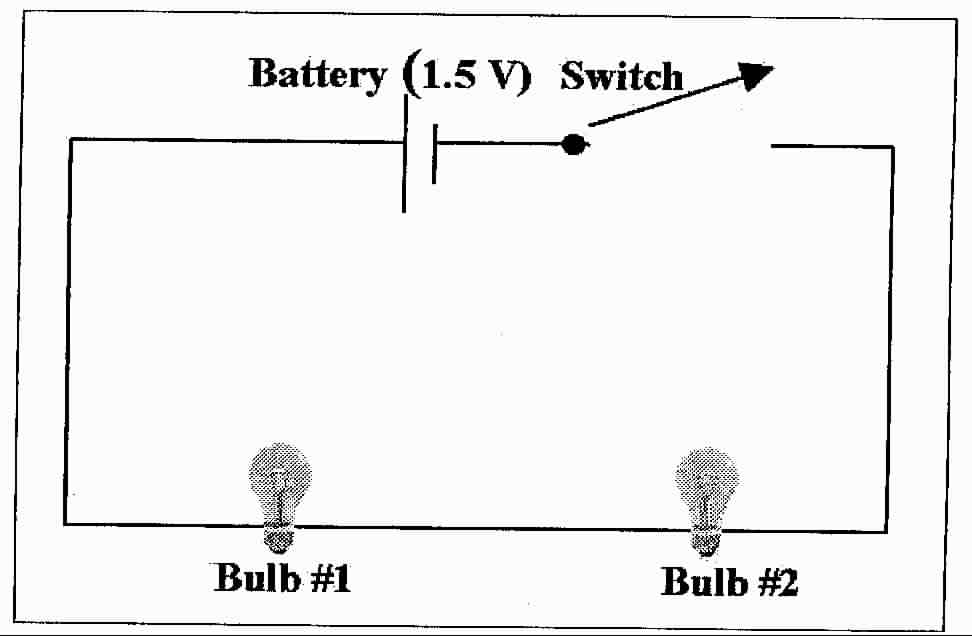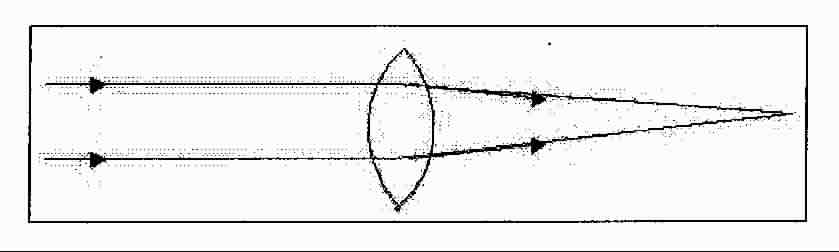Basic Physical Science Concepts Review Test

You are a space traveler and you have entered a solar system around a star called the Sun. You observe the Sun and one of its nine planets, the Earth, along with its satellite, the Moon. They are lined up in the following order.1. Observers on the Earth would see which of the following?

1. full moon
2. lunar eclipse
3. solar eclipse
4. first quarter moon

You decide to spend time exploring this part of the Milky Way Galaxy and place your space ship in a large orbit around this Sun and its planets. About 29 to 30 days later you notice that the Sun, Earth and Moon are lined up in the following order.2. Observers on the Earth would see which of the following?

1. full moon
2. lunar eclipse
3. solar eclipse
4. first quarter moon

3. It is the 4th of July and you and some friends are at the lake. The temperature is 95° F. It is hotter in the summer because

1. the Earth is closer to the Sun
2. the Northern Hemisphere is tilted toward the Sun
3. of an increase in solar activity
4. of changes in the jet stream

4. You are a lunar astronomer and you have observed the Moon as it goes through a lunar month. Of the following, which best explains why the Moon goes through the different phases as seen by an observer on Earth?

1. Earth’s shadow on the lunar surface
2. The rotation of the Moon on its axis
3. The Moon revolves around Earth
4. The Earth’s rotation on its axis

5. As the space traveler in questions 1& 2 you observe that the closest star to the planet that you have named Earth is

1. Alpha Centauri
2. Sirius
3. Polaris (North Star)
4. Sun

6. Of the following, which best describes an object with a definite shape and volume?

1. solid
2. density
3. liquid
4. vapor

7. The force of gravity between the Earth and an object is its ____________.

1. weight
2. mass
3. volume
4. height

8. You determine the mass if 5 pennies to be 12 grams and the volume to be 1.34 millimeters. This mass divided by the volume is equal to 8.96 grams/millimeter. What property of these pennies have you determined?

1. density
2. area
3. weight
4. temperature

9. The amount of matter in an object is its

1. weight
2. mass
3. volume
4. height

10. You are working in a lab and have dissolved 5 grams of sodium chloride (table salt) in 100 ml of water. Of the following, which best describes the product?

1. mixture
2. compound
3. molecule
4. none of the above

11. Match the following compounds to their chemical formula:

H2SO4 a. ammonium hydroxide

NH4OH b. calcium carbonate

CaCO3 c. glucose

C6H12O6 d. sulfuric acid

12. Lemonade tastes sour because it is

1. neutral
2. acidic
3. basic

13. Electrons repel each other and protons repel each other, while a proton and electron attract each other. How does the "Big Rule" of electricity and magnetism explain this phenomenon?

1. like charges repel—opposite charges repel
2. opposite charges attract—like charges repel
3. electrons are neutral and exhibit no force
4. protons are neutral and exhibit no force

13. You touch the red wire of a voltmeter to the anode of a flash light battery, at the same time you touch the black wire to the cathode of the battery. The meter reads 1.5 V. What have you measured?1. resistance
2. current
3. inductance
4. potential difference

14. What type of circuit does this illustration represent?

1. parallel
2. three phase
3. twelve volt
4. series

15. If bulb #2 (from previous circuit in questions #14) is removed

1. bulb #1 will continue to glow with the same brightness.
2. bulb #1 will glow more brightly
3. bulb #1 will no longer glow16. What type of circuit does this illustration represent?

1. parallel
2. three phase
3. twelve volt
4. series

17. I bulb #2 is removed

1. bulb #1 will continue to glow with the same brightness
2. bulb #1 will glow more brightly
3. bulb #1 will no longer glow

18. You observe the following (diagram on next page): As light passes through a convex lens (like a magnifying glass) it converges at on point called a focus or image point. Of the following terms, which one explains this phenomenon?

1. refraction of light
2. reflection of light
3. projection of light
4. transmission of light19. A blue car appears blue because blue light is _______.

1. absorbed
2. reflected
3. projected

20. An object that you see as the color black

1. deflecting all wavelengths of light
2. absorbing all wavelengths of light
3. reflecting all wavelengths of light

21. You are directed to set up an experiment in which you drop, from shoulder height, objects with similar surface areas but different masses, timing how long it takes for each object to hit the floor. Of the following explanations, which best describes your findings?

1. the most dense object hits the ground first
2. the less dense object hits the ground first
3. they will hit the ground at the same time

22. An archer has just released the string on his bow shooting an arrow. Which of the following best describes the energy of the arrow.

1. the arrow has kinetic energy
2. the arrow has no energy
3. the arrow has electrical energy
4. the arrow has elastic potential energy

23. You are an automobile engineer and you must decide what size engine to install in a large luxury car so that is can accelerate as quickly as a small sports car. Which of the following descriptions of Newton’s laws are you most concerned with?

1. force is equal to mass times acceleration
2. for every action there is an equal and opposite reaction
3. an object in motion will continue in motion

24. The freezing point temperature of liquid water is

1. one Celsius degree higher that the melting point of water ice
2. equal to the melting point of water ice
3. a fraction of a Celsius degree lower than the melting point of water
4. 0.0° C, while the melting point of water ice is 1.0° C.

Basic Physical Science Concepts Review Test - KEY

You are a space traveler and you have entered a solar system around a star called the Sun. You observe the Sun and one of its nine planets, the Earth, along with its satellite, the Moon. They are lined up in the following order.1. Observers on the Earth would see which of the following?

1. full moon
2. lunar eclipse
3. solar eclipse
4. first quarter moon

You decide to spend time exploring this part of the Milky Way Galaxy and place your space ship in a large orbit around this Sun and its planets. About 29 to 30 days later you notice that the Sun, Earth and Moon are lined up in the following order.2. Observers on the Earth would see which of the following?

1. full moon
2. lunar eclipse
3. solar eclipse
4. first quarter moon

3. It is the 4th of July and you and some friend are at the lake. The temperature is 95° F. It is hotter in the summer because

1. the Earth is closer to the Sun
2. the Northern Hemisphere is tilted toward the Sun
3. of an increase in solar activity
4. of changes in the jet stream

4. You are a lunar astronomer and you have observed the Moon as it goes through a lunar month. Of the following, which best explains why the Moon goes through the different phases as seem by an observer on Earth?

1. Earth’s shadow on the lunar surface
2. The rotation of the Moon on its axis
3. The Moon revolves around Earth
4. The Earth’s rotation on its axis

5. As the space traveler in questions 1& 2 you observe that the closest star to the planet that you have named Earth is

1. Alpha Centauri
2. Sirius
3. Polaris (North Star)
4. Sun

6. Of the following, which is best describes an object with a definite shape and volume?

1. solid
2. density
3. liquid
4. vapor

7. The force of gravity between the Earth and an object is its ____________.

1. weight
2. mass
3. volume
4. height

8. You determine the mass of 5 pennies to be 12 grams and the volume to be 1.34 millimeters. This mass divided by the volume is equal to 8.96 grams/millimeter. What property of these pennies have you determined?

1. density
2. area
3. weight
4. temperature

9. The amount of matter in an object is its

1. weight
2. mass
3. volume
4. height

10. You are working in a lab and have dissolved 5 grams of sodium chloride (table salt) in 100 ml of water. Of the following, which best describes the product?

1. mixture
2. compound
3. molecule
4. none of the above

11. Match the following compounds to their chemical formula:

d. H2SO4 a. ammonium hydroxide

a. NH4OH b. calcium carbonate

b. CaCO3 c. glucose

c. C6H12O6 d. sulfuric acid

12. Lemonade tastes sour because it is

1. neutral
2. acidic
3. basic

13. Electrons repel each other and protons repel each other, while a proton and electron attract each other. How does the "Big Rule" of electricity and magnetism explain this phenomenon?

1. like charges repel—opposite charges repel
2. opposite charges attract—like charges repel
3. electrons are neutral and exhibit no force
4. protons are neutral and exhibit no force

13. You touch the red wire of a voltmeter to the anode of a flash light battery, at the same time you touch the black wire to the cathode of the battery. The meter reads 1.5 V. What have you measured?1. resistance
2. current
3. inductance
4. potential difference

14. What type of circuit does this illustration represent?

1. parallel
2. three phase
3. twelve volt
4. series

15. If bulb #2 (from previous circuit in questions #14) is removed

1. bulb #1 will continue to glow with the same brightness.
2. bulb #1 will glow more brightly
3. bulb #1 will no longer glow16. What type of circuit does this illustration represent?

1. parallel
2. three phase
3. twelve volt
4. series

17. If bulb #2 is removed

1. bulb #1 will continue to glow with the same brightness
2. bulb #1 will glow more brightly
3. bulb #1 will no longer glow

18. You observe the following (diagram on next page): As light passes through a convex lens (like a magnifying glass) it converges at on point called a focus or image point. Of the following terms, which one explains this phenomenon?

1. refraction of light
2. reflection of light
3. projection of light
4. transmission of light19. A blue car appears blue because blue light is _______.

1. absorbed
2. reflected
3. projected

20. An object that you see as the color black

1. deflecting all wavelengths of light
2. absorbing all wavelengths of light
3. reflecting all wavelengths of light

21. You are directed to set up an experiment in which you drop, from shoulder height, objects with similar surface areas but different masses, timing how long it takes for each object to hit the floor. Of the following explanations, which best describes your findings?

1. the most dense object hits the ground first
2. the less dense object hits the ground first
3. they will hit the ground at the same time

22. An archer has just released the string on his bow shooting an arrow. Which of the following best describes the energy of the arrow.

1. the arrow has kinetic energy
2. the arrow has no energy
3. the arrow has electrical energy
4. the arrow has elastic potential energy

23. You are an automobile engineer and you must decide what size engine to install in a large luxury car so that is can accelerate as quickly as a small sports car. Which of the following descriptions of Newton’s laws are you most concerned with?

1. force is equal to mass times acceleration
2. for every action there is an equal and opposite reaction
3. an object in motion will continue in motion

24. The freezing point temperature of liquid water is

1. one Celsius degree higher that the melting point of water ice
2. equal to the melting point of water ice
3. a fraction of a Celsius degree lower than the melting point of water
4. 0.0° C, while the melting point of water ice is 1.0° C.